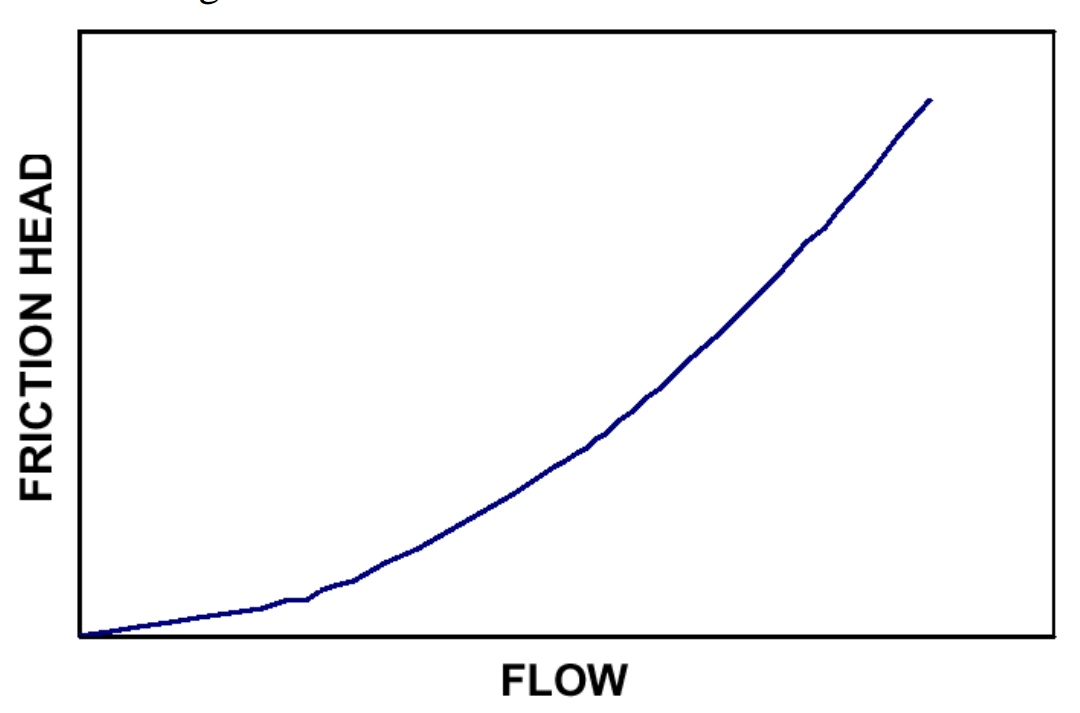### Translate

Affinity laws
Pumping Terms
Effect of Impeller diameter change

### 1. Affinity law

Centrifugal pump is a dynamic device which generates discharge pressure with impeller rotation.

Impeller rotation is directly connected to fluid peripheral velocity and generated head.

Centrifugal pump affinity laws states relation between impeller rotation with Flow rates, Generated head,  Power consumption.

Flow rate [Q] is directly proportional to rotational speed of Impeller [N].
Impeller Diameter [D] is directly proportional to rotational speed of Impeller [D].

Q ∝ N  ;  Q ∝ D

Head [H] is directly proportional to square of impeller rotational speed [N].
Head [H] is directly proportional to square of impeller diameter [D].

H N2 ;  D2

Suction requirement is also increasing in square proportion with increasing in flow.

NPSHR ∝ N²

Power [P] is proportion to cube of impeller rotational speed [N].
Power [P] is proportion to cube of impeller diameter [D].

P N ;  D3

Example : 1

If Pump RPM is 1750 and giving 100 m3/hr discharge flow. If we double Pump RPM then how much flow will be generated

Q1 = 100 M3
Q2 = ?
N1 = RPM1 = 1750
N2 = RPM2 = 3500

Q2 = 200 m3/hr

Example : 2

Pump head is 100 meter on 1750 RPM then how much head generated on 3500 RPM?

H1 = 100 meter
H2 = ?
N1 = RPM1 = 1750
N2 = RPM2 = 3500

H2 = 400 meter

Example : 3

Pump power consumption 5kW on 1750 RPM then how much power consumption will be on 3500 RPM?
P1 = 5 kW
P2 = ?
N1 = RPM1 = 1750
N2 = RPM2 = 3500

P2 = 40 kW

#### Conclusion

When we double pump RPM then
• Head will be 4 Times
• Power consumption will be 8 Times
Pumping Terms

Pump diacharge pressure is required to transfer liquid.

But there are resistance in system called losses.

Losses in pumping system are two types.

1. Static loss or static head
2. Friction loss or Dynamic loss

Static head is difference in height between Highest level of reservoir and Destination.

When pump diacharge liquid in pipes there is already previous pumped liquid present in discharge line.

This previous pumped liquid offer resistance for pump discharge is called Static head loss.

Suppose pump discharge line is larger diameter and 3 times head then pump size then pump will not able to discharge liquid.

We can say this there are only static head loss in system.

Static head loss is not dependent on flow of fluid it is system characteristics.Static head vs flow in centrifugal pump

How to reduce static head loss?

Reduce unnecessary pipe length, valves, elbows, instrumentation etc can reduce static head loss.

When any fluid is flowing through pipes friction is offered by system called Friction head loss.

Fluid is passing through pipes, valves, transmitters etc which adds pressure drop in system due to friction.

There are two frictions in system

This friction result in reduction of flow.

It is available in standard table format for valves fittings and pipes in 100 meter for spicific diameter.

Friction loss is directly proportional to square of flow rate.

###Friction loss vs FlowHow to reduce friction head loss?Use larger diameter pipes.Combine head loss

Most system has combination of Static head loss and Friction head loss

Both losses influence each other.

Let us see.

1. High Static head loss system

Here we can see in this graph...

High static head loss will result in low flow rates.

Low flow rate has less fricrion so friction head loss is lesser.

2. Low Static head loss system

Pumping with low static head loss has high flow rates.

High flow rates creates more friction with pipe walls and fluid layers.

Friction loss is low at low flow rate.
Friction loss is higher at high flow rate.

### Effect of Impeller diameter change.

Over sized pump has more power consumption. Power reduction may possible with 2 methods

Installation of VFD : Variable frequency drive.
Trimming impeller size

Impeller trimming result into reduction of pump internal peripheral velocity. Reduction in pump impeller diameter will follow Affinity law of pump.

Flow rate [Q] is directly proportional to rotational diameter of Impeller

Q ∝ D

Head is directly proportional to square of impeller diameter.

D2

Power P is proportion to cube of impeller diameter.

D3

Impeller diameter reduction is limited to 25% of impeller size. [ 75% of impeller size must be available]

75% size impeller will result in head reduction up to 50%.

Further reduction will not maintain NPSH.